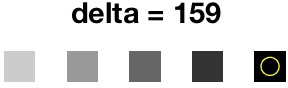### Table of Contents

Maximally Stable Extremal Regions (MSER) is a feature detector; Like the SIFT detector, the MSER algorithm extracts from an image I a number of co-variant regions, called MSERs. An MSER is a stable connected component of some level sets of the image I. Optionally, elliptical frames are attached to the MSERs by fitting ellipses to the regions. For a more in-depth explanation of the MSER detector, see our API reference for MSER

# Extracting MSERs

Each MSERs can be identified uniquely by (at least) one of its pixels x, as the connected component of the level set at level I(x) which contains x. Such a pixel is called seed of the region.

To demonstrate the usage of the MATLAB command vl_mser we open MATLAB and load a test image

pfx = fullfile(vl_root,'data','spots.jpg') ;
I = imread(pfx) ;
image(I) ;A test image.

We then convert the image to a format that is suitable for the vl_mser command.

I = uint8(rgb2gray(I)) ;


We compute the region seeds and the elliptical frames by

[r,f] = vl_mser(I,'MinDiversity',0.7,...
'MaxVariation',0.2,...
'Delta',10) ;


We plot the region frames by

f = vl_ertr(f) ;
vl_plotframe(f) ;


vl_ertr transposes the elliptical frame and is required here because the vl_mser code assumes that the row index is the first index, but the normal image convention assumes that this is the x (column) index.

Plotting the MSERs themselves is a bit more involved as they have arbitrary shape. To this end, we exploit two functions: vl_erfill, which, given an image and a region seed, returns a list of the pixels belonging to that region, and the MATLAB built-in contour, which draws the contour lines of a function. We start by

M = zeros(size(I)) ;
for x=r'
s = vl_erfill(I,x) ;
M(s) = M(s) + 1;
end


which computes a matrix M whose value are equal to the number of overlapping extremal regions. Next, we use M and contour to display the region boundaries:

figure(2) ;
clf ; imagesc(I) ; hold on ; axis equal off; colormap gray ;
[c,h]=contour(M,(0:max(M(:)))+.5) ;
set(h,'color','y','linewidth',3) ;Extracted MSERs (left) and fitted ellipses (right).

# MSER parameters

In the original formulation, MSERs are controlled by a single parameter Δ, which controls how the stability is calculated. Its effect is shown in the figure below.Effect of Δ. We start with a synthetic image which has an intensity profile as shown. The bumps have heights equal to 32, 64, 96, 128 and 160. As we increase Δ, fewer and fewer regions are detected until finally at Δ=160 there is no region R which is stable at R(+Δ).

The stability of an extremal region R is the inverse of the relative area variation of the region R when the intensity level is increased by Δ. Formally, the variation is defined as:

|R(+Δ) - R|
-----------
|R|


where |R| denotes the area of the extremal region R, R(+Δ) is the extremal region +Δ levels up which contains R and |R(+Δ) - R| is the area difference of the two regions.

A stable region has a small variation. The algorithm finds regions which are "maximally stable", meaning that they have a lower variation than the regions one level below or above. Note that due to the discrete nature of the image, the region below / above may be coincident with the actual region, in which case the region is still deemed maximal.

However, even if an extremal region is maximally stable, it might be rejected if:

• it is too big (see the parameter MaxArea);
• it is too small (see the parameter MinArea);
• it is too unstable (see the parameter MaxVariation);
• it is too similar to its parent MSER (see the parameter MinDiversity).

By default, MSERs are extracted for both dark-on-bright regions and bright-on-dark regions. To control this, parmeters BrightOnDark and DarkOnBright which take values 0 or 1 to enable or disable the regions. For example:

[r,f] = vl_mser(I,'MinDiversity',0.7,...
'MaxVariation',0.2,...
'Delta',10,...
'BrightOnDark',1,'DarkOnBright',0) ;


computes the regions in green in the figure below.Extracted MSERs (left) and fitted ellipses (right) for both bright-on-dark (green) and dark-on-bright (yellow).

# Conventions

As mentioned in the introduction, vl_mser uses matrix indices as image coordinates. Compared to the usual MATLAB convention for images, this means that the x and y axis are swapped (this has been done to make the convention consistent with images with three or more dimensions). Thus the frames computed by the program may need to be "transposed" as in:

[r,f] = vl_mser(I) ;
f = vl_ertr(f) ;


On the other hand, the region seeds r are already in row major format, which is the standard MATLAB format for pixel indices.

Instead of transposing the frames, one can start by transposing the image. In this case, the frames f have the standard image convention, but the region seeds are in column-major format and may need to be "transposed" as in:

[r,f] = vl_mser(I') ;
[i,j] = sub2ind(size(I'),r) ;
r  = ind2sub(size(I),j,i) ;


The command line utility mser uses the normal image convention (because images are rasterized in column-major order). Therefore the image frames are in the standard format, and the region seeds are in column major format.

In order to convert from the command line utility convention to the MATLAB convention one needs also to recall that MATLAB coordinates starts from (1,1), but the command line utility uses the more common convention (0,0). For instance, let the files image.frame and image.seed contain the feature frames and seeds in ASCII format as generated by the command line utility. Then

r_ = load('image.seed')' + 1 ;
f_ = load('image.frame')' ;
f_(1:2,:) = f_(1:2,:) + 1 ;
[r,f] = vl_mser(I') ; % notice the transpose


produces identical (up to numerical noise) region seeds r and r_ and frames f and f_.